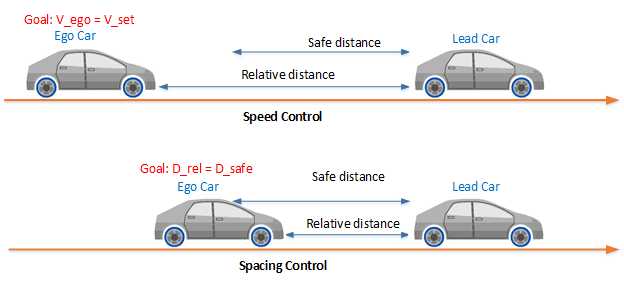Simulate adaptive cruise control using model predictive controller

• Library:
• Model Predictive Control Toolbox / Automated Driving

•## Description

The Adaptive Cruise Control System block simulates an adaptive cruise control (ACC) system that tracks a set velocity and maintains a safe distance from a lead vehicle by adjusting the longitudinal acceleration of an ego vehicle. The block computes optimal control actions while satisfying safe distance, velocity, and acceleration constraints using model predictive control (MPC).To customize your controller, for example to use advanced MPC features or modify controller initial conditions, click .

## Ports

### Input

expand all

Ego vehicle velocity setpoint in m/s. When there is no lead vehicle, the controller tracks this velocity.

Safe time gap in seconds between the lead vehicle and the ego vehicle. This time gap is used to calculate the minimum safe following distance constraint. For more information, see Safe Following Distance.

Ego vehicle velocity in m/s.

Distance in meters between lead vehicle and ego vehicle. To calculate this signal, subtract the ego vehicle position from the lead vehicle position.

Velocity difference in meters per second between lead vehicle and ego vehicle. To calculate this signal, subtract the ego vehicle velocity from the lead vehicle velocity.

Minimum ego vehicle longitudinal acceleration constraint in m/s2. Use this input port when the minimum acceleration varies at run time.

#### Dependencies

To enable this port, select Use external source for the Minimum longitudinal acceleration parameter.

Maximum ego vehicle longitudinal acceleration constraint in m/s2. Use this input port when the maximum acceleration varies at run time.

#### Dependencies

To enable this port, select Use external source for the Maximum longitudinal acceleration parameter.

Controller optimization enable signal. When this signal is:

• Nonzero, the controller performs optimization calculations and generates a Longitudinal acceleration control signal.

• Zero, the controller does not perform optimization calculations. In this case, the Longitudinal acceleration output signal remains at the value it had when the optimization was disabled. The controller continues to update its internal state estimates.

#### Dependencies

To enable this port, select the Use external signal to enable or disable optimization parameter.

Actual longitudinal acceleration in m/s2 applied to the ego vehicle. The controller uses this signal to estimate the ego vehicle model states. Use this input port when the control signal applied to the ego vehicle does not match the optimal control signal computed by the model predictive controller. This mismatch can occur when, for example:

• The Adaptive Cruise Control System is not the active controller. Maintaining an accurate state estimate when the controller is not active prevents bumps in the control signal when the controller becomes active.

• The acceleration actuator fails and does not provide the correct control signal to the ego vehicle.

#### Dependencies

To enable this port, select the Use external control signal for bumpless transfer between ACC and other controllers parameter.

### Output

expand all

Acceleration control signal in m/s2 generated by the controller.

## Parameters

expand all

### Parameters Tab

Ego Vehicle Model

The linear model from the ego vehicle longitudinal acceleration to its longitudinal velocity, specified as an LTI model or a linear System Identification Toolbox model. The controller creates its internal predictive model by augmenting the ego vehicle dynamic model.

Initial velocity in m/s of the ego vehicle model, which can differ from the actual ego vehicle initial velocity.

This value is used to configure the initial conditions of the model predictive controller. For more information, see Initial Conditions.

Minimum spacing in meters between the lead vehicle and the ego vehicle. This value corresponds to the target relative distance between the ego and lead vehicles when the ego vehicle velocity is zero.

This value is used to calculate the:

Maximum ego vehicle longitudinal velocity in m/s.

Minimum ego vehicle longitudinal acceleration constraint in m/s2.

If the minimum acceleration varies over time, add the Minimum longitudinal acceleration input port to the block by selecting Use external source.

Maximum ego vehicle longitudinal acceleration constraint in m/s2.

If the maximum acceleration varies over time, add the Maximum longitudinal acceleration input port to the block by selecting Use external source.

Model Predictive Controller Settings

Controller sample time in seconds.

Controller prediction horizon steps. The controller prediction time is the product of the sample time and the prediction horizon.

Closed-loop controller performance. The default parameter value provides a balanced controller design. Specifying a:

• Smaller value produces a more robust controller with smoother control actions.

• Larger value produces a more aggressive controller with a faster response time.

When you modify this parameter, the change is applied to the controller immediately.

### Block Tab

Configure the controller to apply a suboptimal solution after a specified maximum number of iterations, which guarantees the worst-case execution time for your controller.

#### Dependencies

After selecting this parameter, specify the Maximum iteration number parameter.

Maximum number of controller optimization iterations.

#### Dependencies

To enable this parameter, select the Use suboptimal solution parameter.

To add the Enable optimization input port to the block, select this parameter.

Select this parameter to add the External control signal input port to the block.

Generate a custom ACC subsystem, which you can modify for your application. The configuration data for the custom controller is exported to the MATLAB® workspace as a structure.

You can modify the custom controller subsystem to:

• Modify default MPC settings or use advanced MPC features.

• Modify the default controller initial conditions.

• Use different application settings, such as a custom safe following distance definition.

expand all